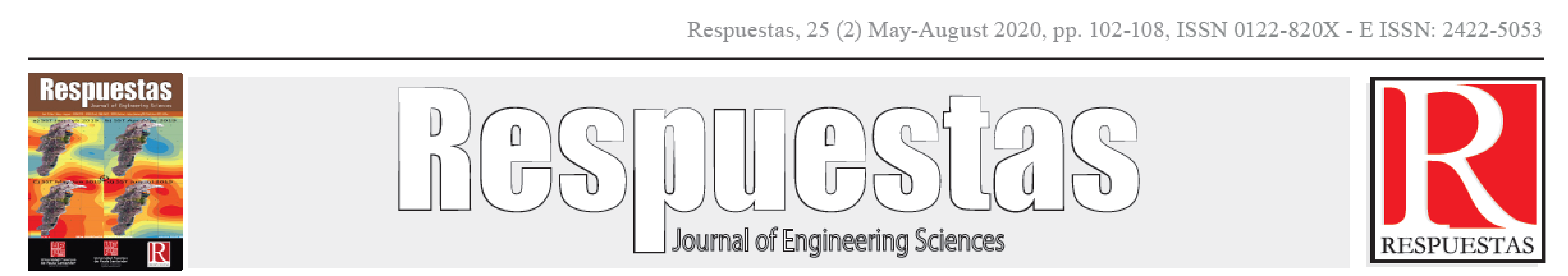Articulo Originalhttps://doi.org/10.22463/0122820X.2819

### Mathematical model of the aquatic phase in the population growth dynamics of Aedes aegypti contaminated with Wolbachia

#### Modelo matemático de la fase acuática en la dinámica de crecimiento de la población de Aedes aegypti contaminada con Wolbachia

##### Eduardo Ibargüen-Mondragón* Jessica Marcela-Montoya* Mawency Vergel-Ortega*

*Department of Mathematics and Statistics edbargun@udenar.edu.co . Universidad de Nariño, Pasto, Nariño, Colombia.

*Department of Mathematics and Statistics jmontoya18@cue.edu.co . Universidad Alexander Von Humboldt, Armenia, Quindío, Colombia.

*Department of Mathematics and Statistics mawency@ufps.edu.co. . Universidad Francisco de Paula Santander, Cúcuta, Norte de Santander, Colombia.

How to cite: E. Ibargüen-Mondragón, J.M. Montoya, M. Vergel-Ortega, “Mathematical model of the aquatic phase in the population growth dynamics of Aedes aegypti contaminated with Wolbachia”. Respuestas, vol. 25, no. 2, pp. 102-108, 2020.

© Peer review is the responsibility of the Universidad Francisco de Paula Santander. This is an article under the license CC BY-NC 4.0.Approved: April 5, 2020.

Abstract

Dengue is an infectious disease of global public health significance and is a leading cause of death. A study focusing on the aquatic phase (egg, larva and pupa) of the Aedes aegypti mosquito, with and without Wolbachia, is being conducted. A mathematical model is formulated, the results suggest that the intrinsic ovoposition rate is the parameter that most affects the non-trivial equilibrium solution. As a conclusion, it is suggested that, under certain conditions, the reproduction of Wolbachia-contaminated mosquitoes in the aquatic phase is small compared to the reproduction of wild mosquitoes, which could affect the success of the control strategy.

Keywords

Aedes aegypti, water phase, mathematical modeling, Wolbachia, ordinary differential equations.

Resumen:

El dengue es una enfermedad infecciosa de importancia para la salud pública mundial y es una de las principales causas de muerte. Se realiza un estudio centrado en la fase acuática (huevo, larva y pupa) del mosquito Aedes aegypti, con y sin Wolbachia. Se formula un modelo matemático, cuyos resultados sugieren que la tasa de ovoposición intrínseca es el parámetro que más afecta a la solución de equilibrio no trivial. Como conclusión, se sugiere que, bajo ciertas condiciones, la reproducción de los mosquitos contaminados con Wolbachia en la fase acuática es pequeña en comparación con la reproducción de los mosquitos salvajes, lo que podría afectar al éxito de la estrategia de control.

Palabras Claves

Aedes aegypti, fase acuática, modelización matemática, Wolbachia, ecuaciones diferenciales ordinarias.

Introduction

The is an extensive literature on mathematical modeling of the population dynamics of the aedes aegypti mosquito that considers both the aquatic and aerial phases of the mosquito [1-9]. However, most references to aquatic phase modeling consider a population group that compacts eggs, larvae and pupae into a single dynamic variable. This form of modeling does not consider factors such as the percentage of eggs that develop into larvae or the percentage of larvae that develop into pupae. Several studies have shown that the aquatic phase is fundamental, not only in the growth process of the vector's population, but also in the epidemiology of mosquito-borne diseases. Moreover, around the world, the main strategies for controlling the mosquito population, such as mosquito breeding ground elimination campaigns, focus on reducing the population of the mosquito in its aquatic phase. However, there are other control strategies such as biological ones that contribute significantly to the reduction of the basic breeding number of the vector. All this motivates us to formulate a mathematical model that describes the dynamics of the aquatic phase explicitly considering three population groups in the aquatic phase.

Methodology

Mathematical model –

The dynamics consider natural (wild) mosquito populations and mosquitos contaminated with Wolbachia. For each group, the life of mosquitoes is defined and divided into the following stages: eggs, larvae and pupae. The following variables are defined on the basis of the above:

Aquatic phase for wild or natural mosquitoes:

E(t) is the amount of eggs in time t.

L(t) is the amount of larvae in time t.

P(t) is the amount of pupae in time t.

Aquatic phase for mosquitoes contaminated with Wolbachia:

a) Ew (t) is the amount of eggs with Wolbachia in time t.

b) Lw (t) is the amount of larvae with Wolbachia in time t.

c) Pw (t) is the amount of pupae with Wolbachia over time t.

The growth dynamics for the wild mosquito egg population is modeled by the following differential equation (1):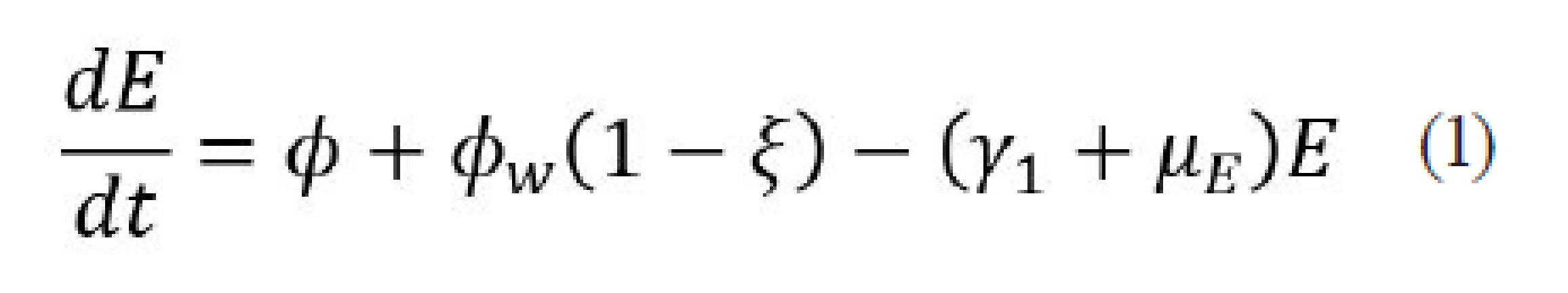Where (ϕ) is the intrinsic ovoposition rate, (ϕw=ϕa) is the ovoposition rate of mosquitoes with Wolbachia, with 0< a< 1 a rate of adjustment that arises because not all the eggs of adult females with Wolbachia hatch, (ξ) is the probability of the Wolbachia bacteria being transferred vertically, (γ1) is the per capita rate of transformation of the egg to the larval stage, (μE) is the natural death rate of eggs.

The dynamics for eggs with Wolbachia is described by the following differential equation (2):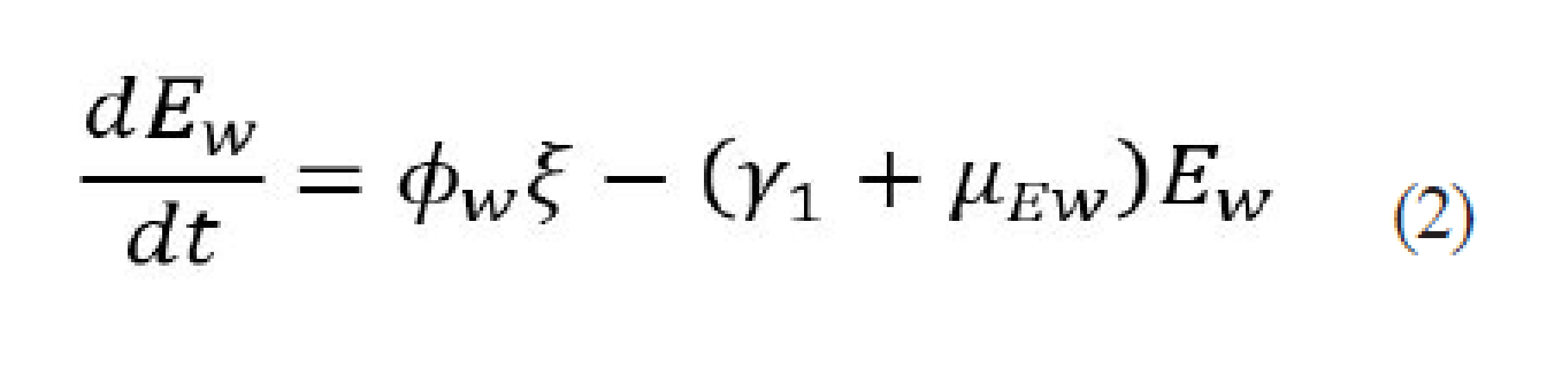Where (μEw) is the natural death rate of eggs with Wolbachia.

The dynamics for wild mosquito larvae is modeled by Equation (3):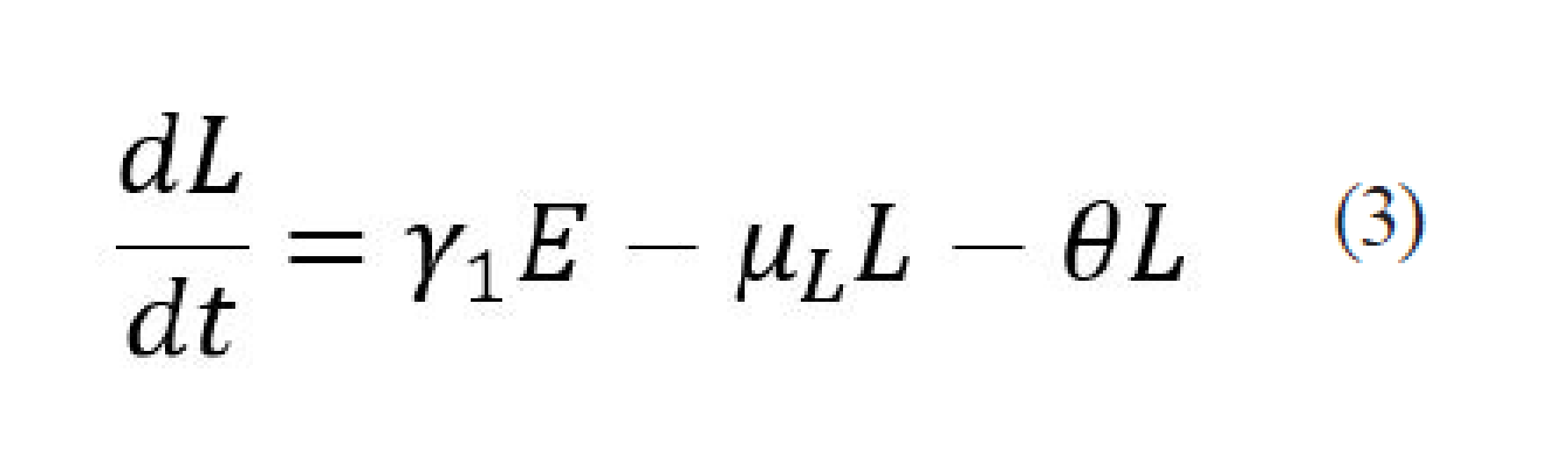Where (θ) is the per capita rate of transformation of the larva to the pupal stage.

The dynamics for Wolbachia-contaminated mosquito larvae is modeled by Equation (4):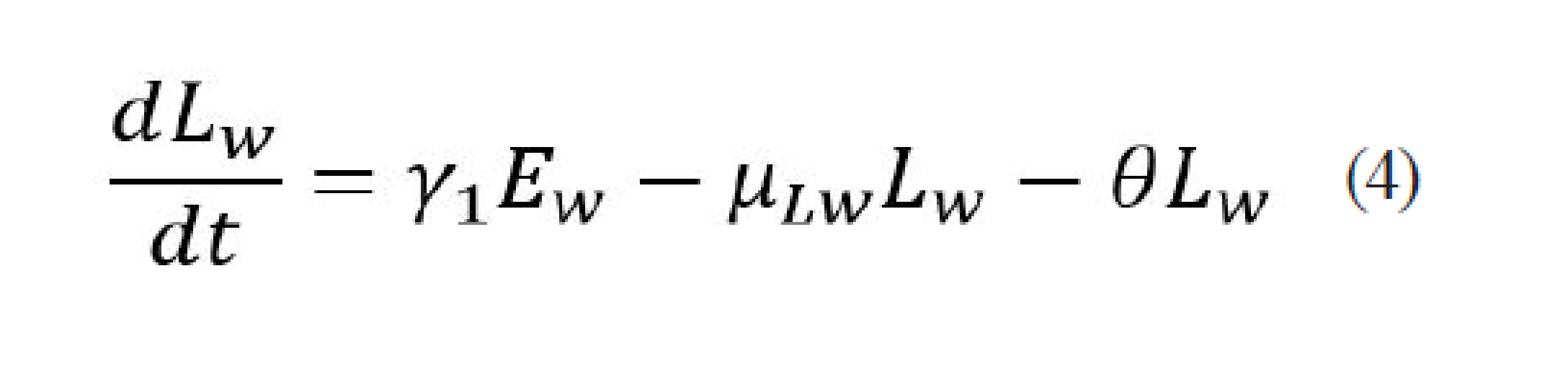Where (μLw) is the natural death rate of the larvae with Wolbachia.

The dynamics for wild mosquito pupae is modeled by Equation (5):Where (μPw) is the natural death rate of Wolbachia pupae.

Be x(t) = (E(t), Ew (t), L(t) ,Lw (t), P(t), Pw (t)T a solution of the system of equations (1) to (6), then x Є Ω, where: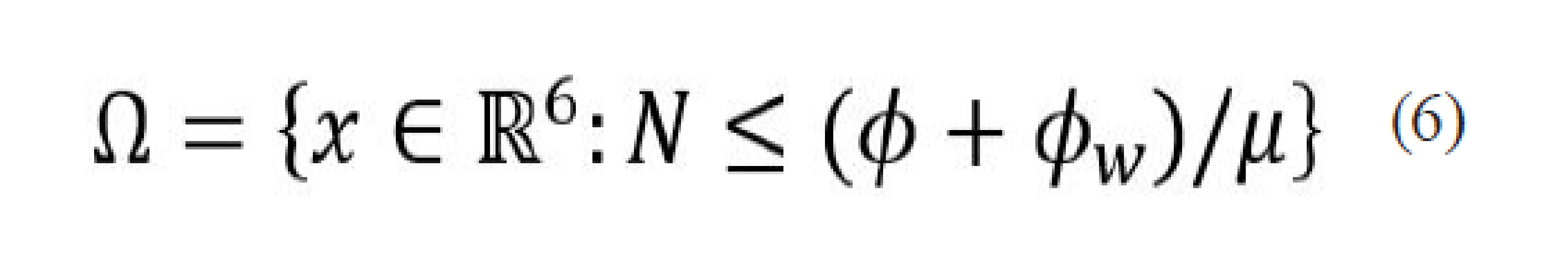Where N=E+Ew+L+Lw+P+Pw and μ = min {μE, μEw, μL, μLw, μP, μPw}, the whole Ω defined in (7) is the set of biological interest since any solution of the system (1)-(6) which starts in Ω stays there for everything t ≥ 0. The system (1)-(6) has a single globally asymptotically stable equilibrium solution given by Equation (8):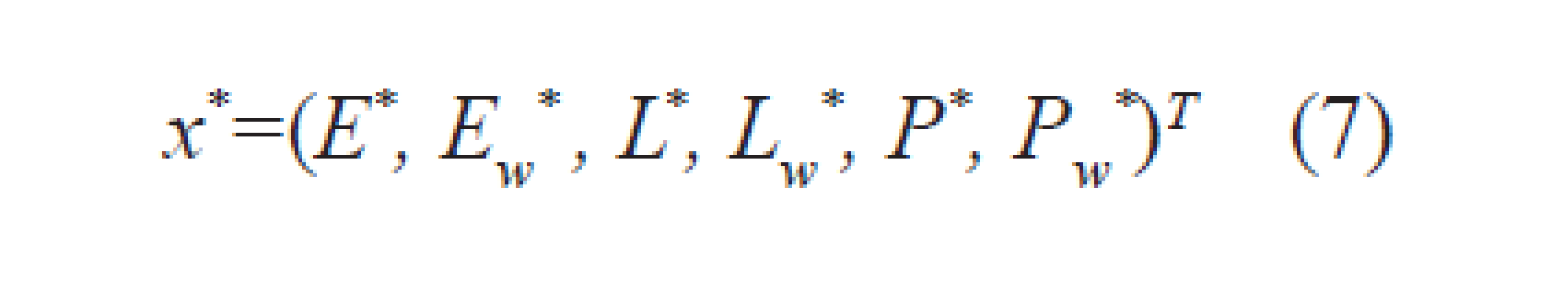Where,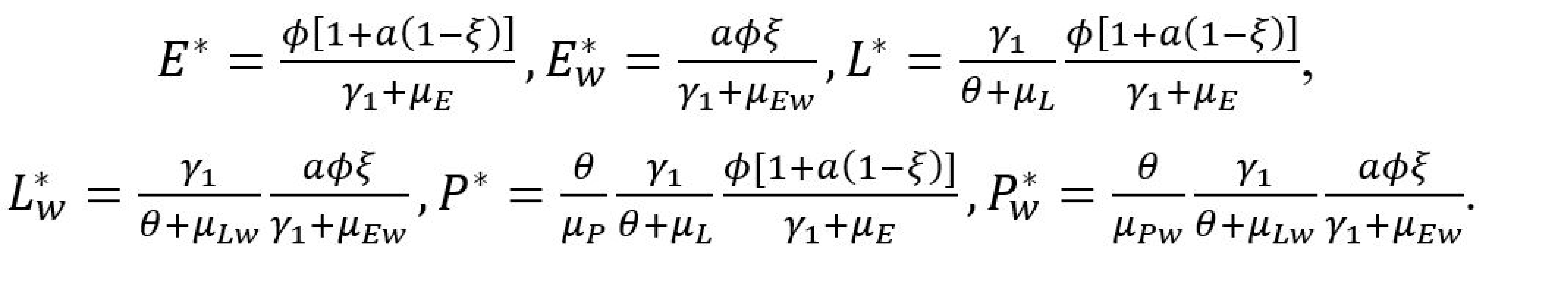The above implies that any solution of the system (1)-(6) in Ω tends to approach x^* equilibrium as time t grows.

Result and Discussion

Numerical simulations

A parameter sensitivity analysis similar to that performed by other authors [8-10] verifies the intrinsic ovoposition rate, (ϕ), is the parameter that most influences the magnitude of the equilibrium solution (x^*). According to Equation (8) it can be seen that all the populations are directly proportional (ϕ), which implies that (x^*=ϕX) where (X) is a vector that depends on the other parameters. On the other hand, after (ϕ), the parameters that most affect the sensitivity of (x^*) are the rate of adjustment (a), and the probability of vertical transmission of bacteria (ξ). Following the order is the per capita rate of transformation of the egg to the larval stage (γ_1) and the per capita rate of transformation of the larva to the pupal stage (θ). Finally, at the last level are the mortality rates. There are many climatic variables (temperature, rainfall and humidity, among others) focuses on temperature or precipitation. So, Table 1, presents a range of estimated parameters based on temperature changes .Table I: Description of parameters.

The parameter ranges (ϕ,ξ,θ,γ_1,μ_E,μ_L,μ_P) were estimated using functions adjusted to data from Chiang Mia province in Thailand . Since no references were found to the values of (a,ϕw,μEw,μLw and μPw) were considered the broadest ranges. So, Figure 1, shows the plots of eggs, larvae and pupae, uncontaminated and contaminated with Wolbachia according to the ovoposition rate (ϕ), as it is observed for each egg contaminated with Wolbachia there are 300 eggs without Wolbachia, the same relation is presented with the larvae and pupae. Figure 2, shows the plots of eggs, larvae and pupae, uncontaminated and contaminated with Wolbachia as a function of the rate of adjustment (a), it is observed that under the set of reference values (Table 1) the variation of the population of eggs, larvae and pupae with Wolbachia is very small in relation to the population of eggs, larvae and pupae without Wolbachia. Figure 3, shows the graphs of eggs, larvae and pupae, uncontaminated and contaminated with Wolbachia as a function of the ovoposition rate (ϕ) and the adjustment rate (a), it is observed that the yield in all populations increased in relation to the yields with respect to each parameter.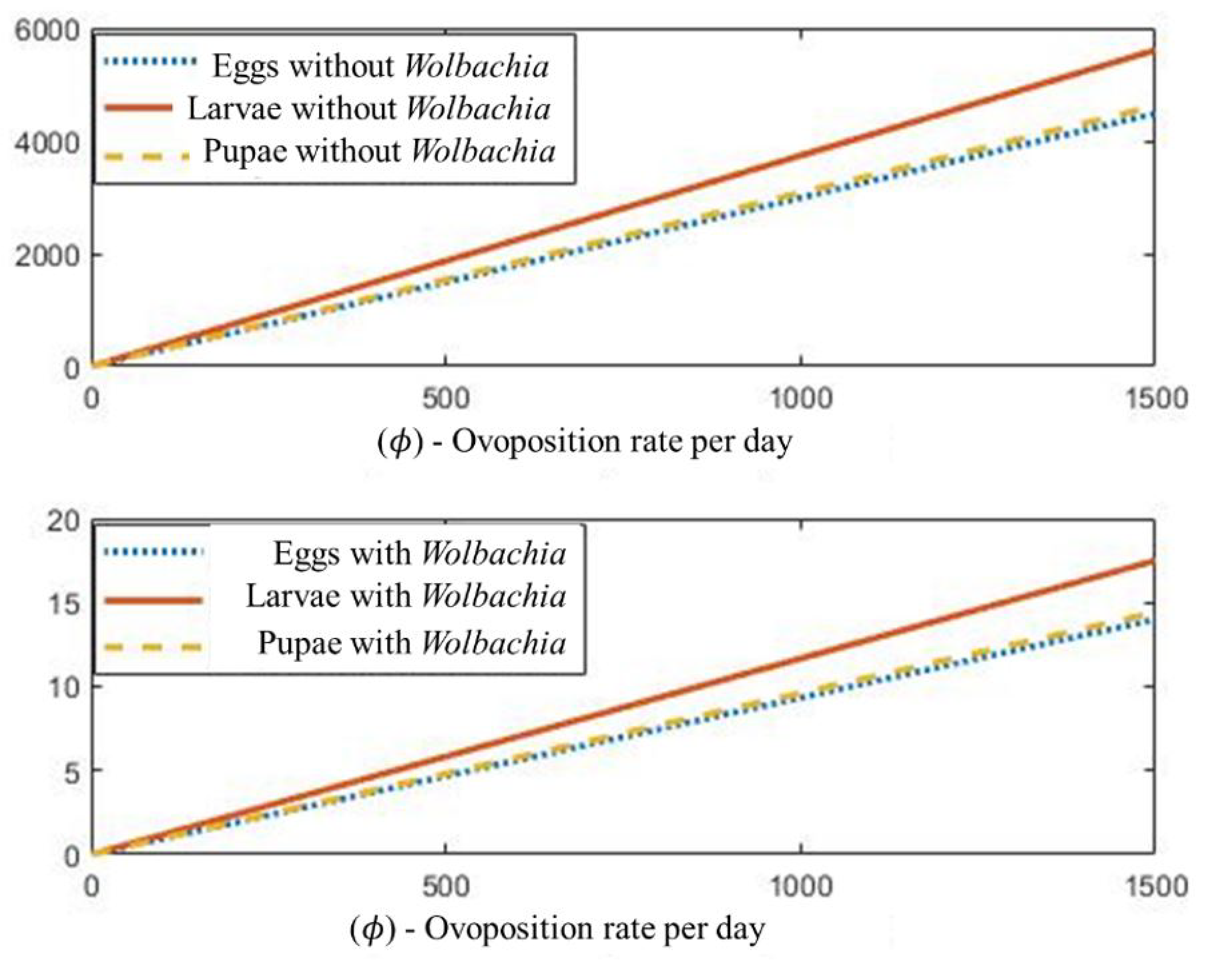Figure 1. a) Population growth of eggs, larvae and pupae, without Wolbachia, with respect to the ovoposition rate (ϕ), taking the range of (ϕ) and the fixed reference values for the remaining parameters. b) Population growth of eggs, larvae and pupae, with Wolbachia, with respect to the ovoposition rate (ϕ), taking the range of (ϕ) and the fixed reference values for the remaining parameters.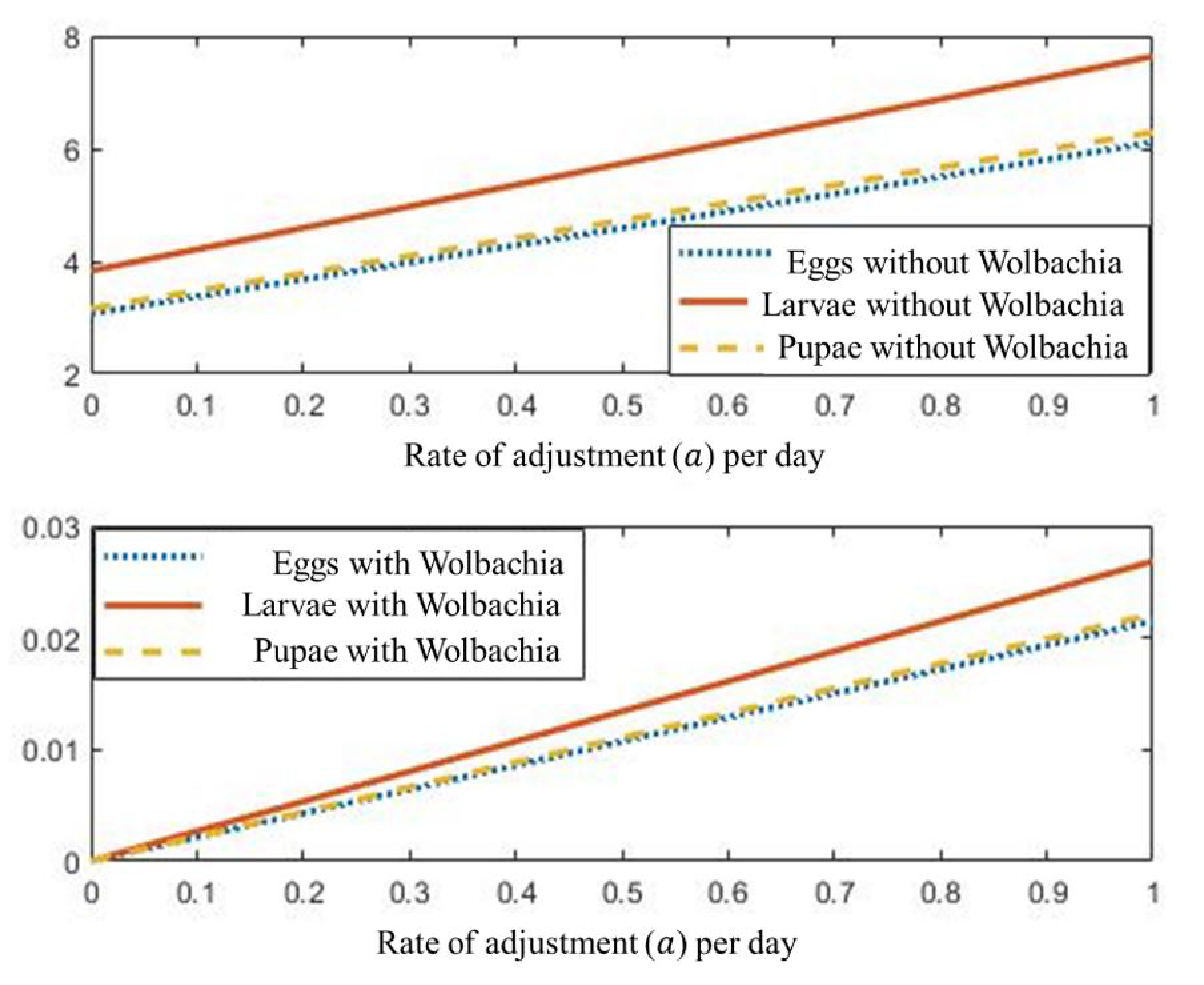Figure 2. a) Growth of the population of eggs, larvae and pupae, without Wolbachia, respect to the adjustment rate (a), taking the range of the adjustment rate (a) and the reference values, fixed for the rest of parameters. b) Growth of the population of eggs, larvae and pupae, with Wolbachia, respect to the adjustment rate (a), taking the range of the adjustment rate (a) and the reference values, fixed for the rest of parameters.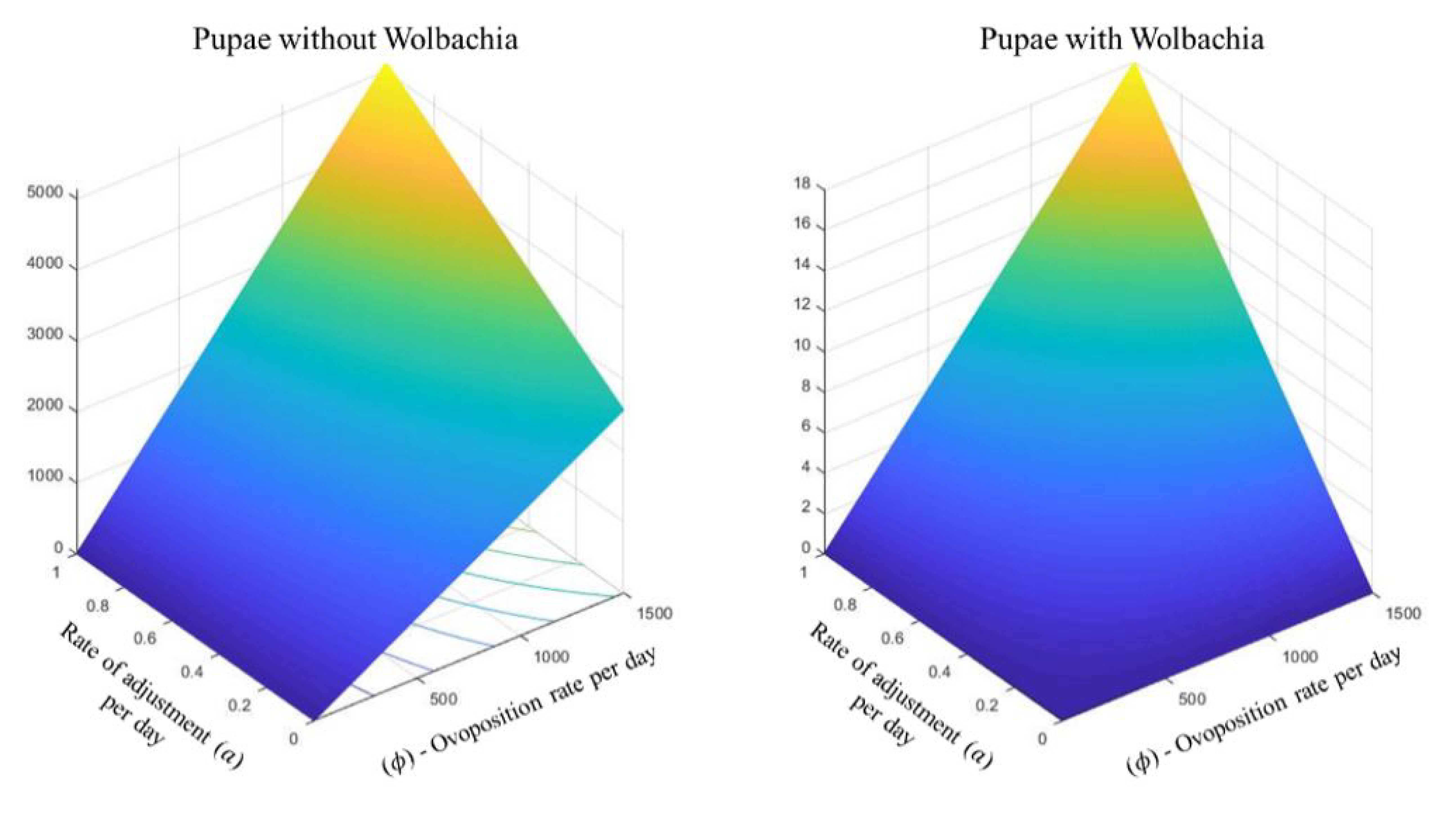Figure 3: a) Growth of the population of pupae without Wolbachia, respect to the adjustment rate (a) and the ovoposition rate (ϕ).
Figure 3: b) Growth of the population of pupae with Wolbachia, respect to the adjustment rate (a) and the ovoposition rate (ϕ).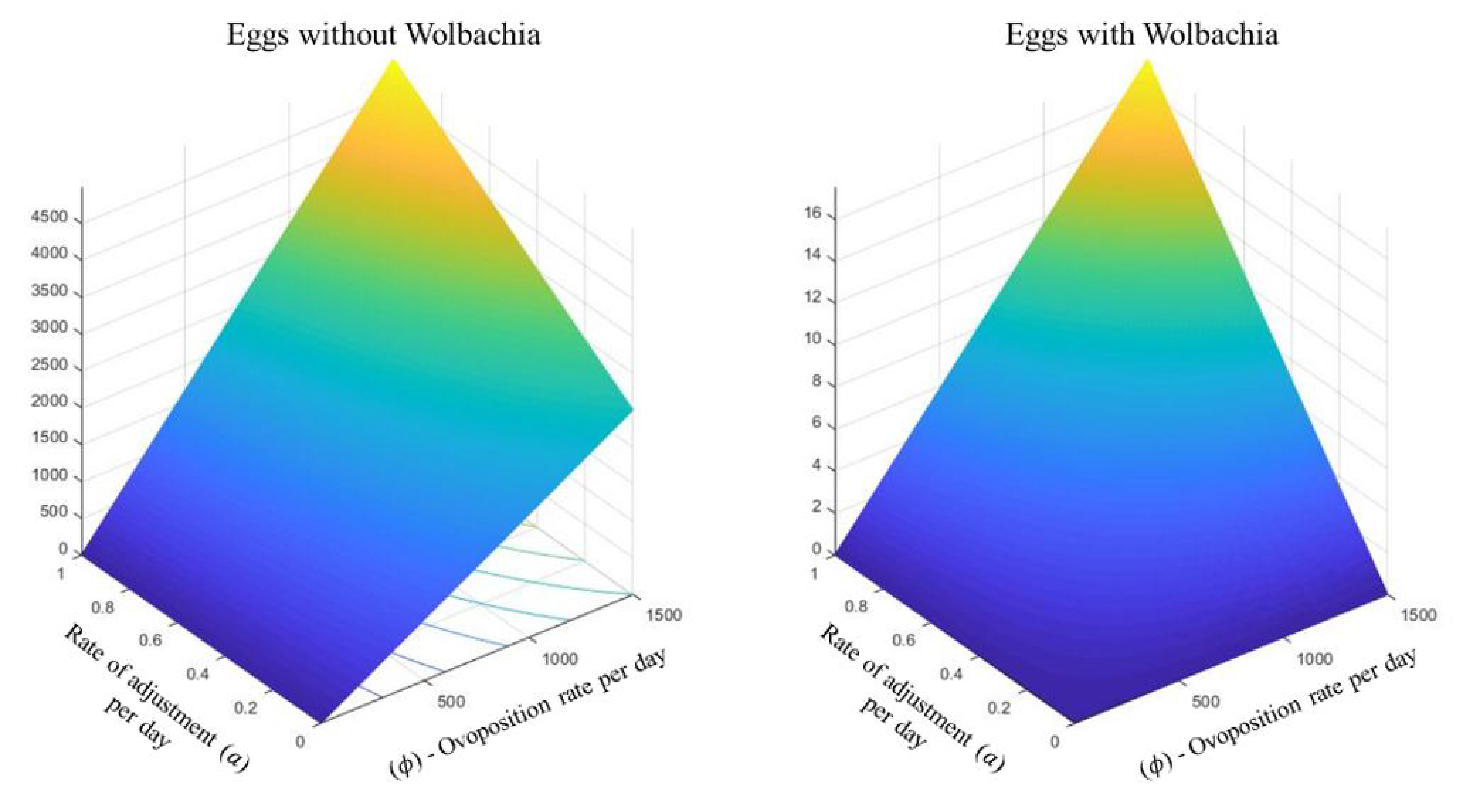Figure 3: c) Growth of the population of eggs without Wolbachia, respect to the adjustment rate (a) and the ovoposition rate (ϕ).
Figure 3: d) Growth of the population of eggs with Wolbachia, respect to the adjustment rate (a) and the ovoposition rate (ϕ).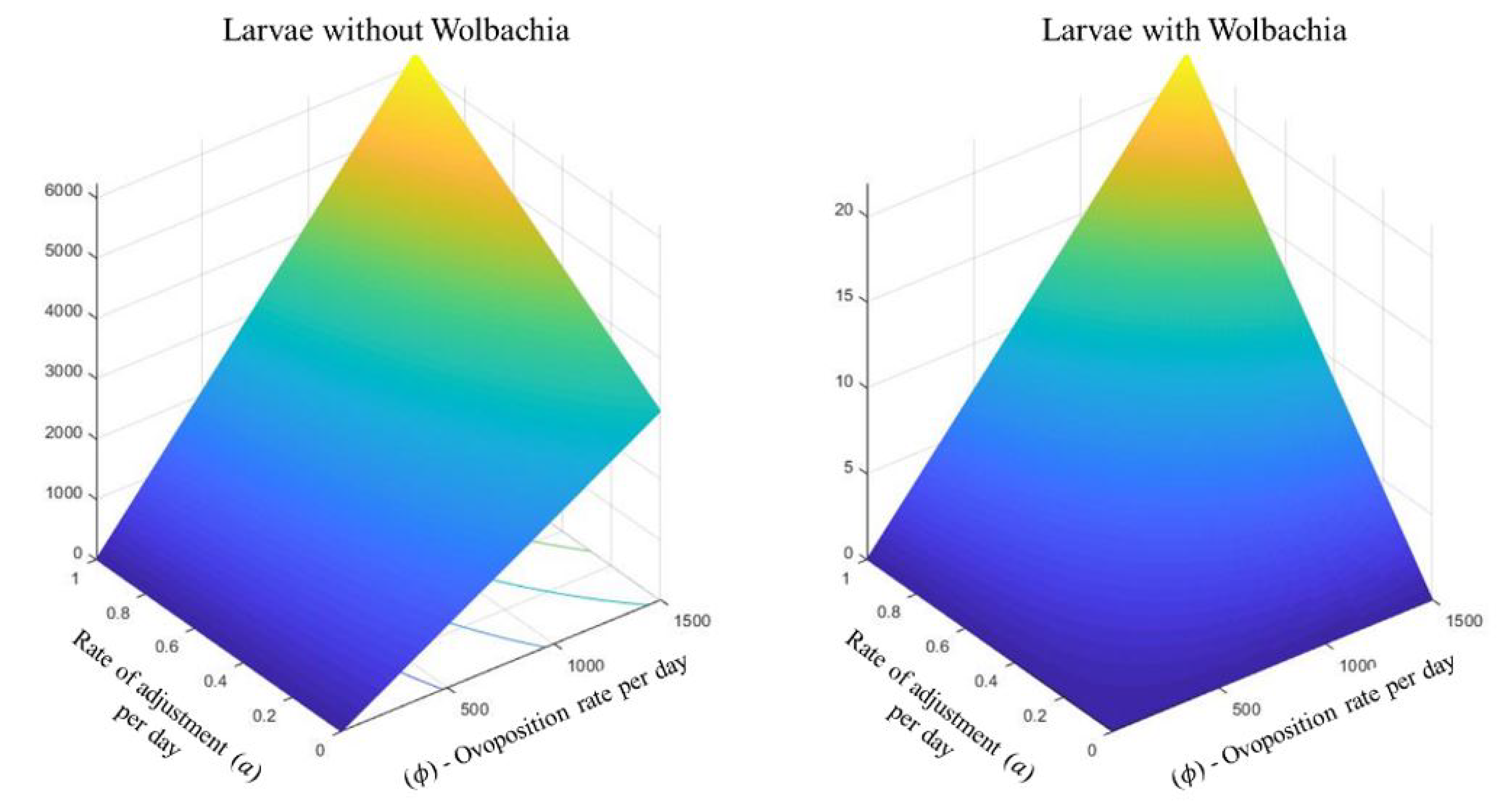Figure 3: e) Growth of the population of larvae without Wolbachia, respect to the adjustment rate (a) and the ovoposition rate (ϕ).
Figure 3: f) Growth of the population of larvae with Wolbachia, respect to the adjustment rate (a) and the ovoposition rate (ϕ).

Figure 3: Growth of the population of eggs, larvae and pupae, with and without Wolbachia, with respect to the adjustment rate (a) and the ovoposition rate (ϕ).

Conclusion

Within the dynamic spread of the Aedes aegypti mosquito and transmission of Dengue, the water phase is of great importance. In fact, the most widely used strategies to control the spread of the mosquito are aimed at reducing the population of eggs, larvae and pupae (larvicides, insecticides, elimination of breeding sites). In recent years, strategies based on biological control have been implemented, in particular the replacement of wild mosquitoes by Wolbachia-infected mosquitoes is a strategy that is gaining ground among all biological control strategies. Although the method concentrates on the aerial phase of the mosquito, the aquatic phase involves the breeding of Wolbachia-contaminated eggs, larvae and pupae, which will later develop into contaminated adult mosquitoes. In this sense, the amount of contaminated mosquitoes that pass from the aquatic phase to the aerial phase contributes directly to the success of the strategy. The results of this work suggest that under certain conditions the breeding of Wolbachiacontaminated mosquitoes in the aquatic phase is very small compared to the breeding of wild mosquitoes. This may affect the success of the control strategy.

Acknowledgements

E. Ibargüen-Mondragón thanks the support of Project N° 114-19/10/2017 (VIIS-UDENAR).

References

 L. Esteva y C. Vargas 1998 “Analysis of a dengue disease transmission model”. Mathematical biosciences Vol 150, No 2, pp 131-151.

 L. Esteva y H. M Yang 2005 “Mathematical model to assess the control of Aedes aegypti mosquitoes by the sterile insect technique”, Mathematical biosciences Vol 198, No 2, pp 132- 147.

 R. C. Thomé, H. M. Yang y L. Esteva 2010 “Optimal control of Aedes aegypti mosquitoes by the sterile insect technique and insecticide”. Mathematical Biosciences, Vol 223, No 1, pp 12- 23.

 S. T. Pinho et al. 2010 “Modelling the dynamics of dengue real epidemics”, Philosophical Transactions of the Royal Society A, Vol 368, No 1933, pp 5679-5693.

 C. P. Ferreira 2020 “Aedes aegypti and Wolbachia interaction: population persistence in an environment changing”, Theoretical Ecology, Vol 13, pp 1-12.

 R. Taghikhani y A. B. Gumel 2018“Mathematics of dengue transmission dynamics: Roles of vector vertical transmission and temperature fluctuations”, Infectious Disease Modelling Vol 3, pp 266-292.

 H. M. Yang y C. P. Ferreira 2008 “Assessing the effects of vector control on dengue transmission”. Applied Mathematics and Computation, Vol 19, No 1, pp 401-413.

 D. Cardona-Salgado et al. 2020 “Wolbachiabased biocontrol for dengue reduction using dynamic optimization approach”. Applied Mathematical Modelling, Vol 82, pp 125-149.

 H. S. Rodrigues, M. T. Monteiro y D. F. Torres 2013 “Sensitivity analysis in a dengue epidemiological model”, Conference Papers in Science, Vol 2013, Art. ID 721406, 7 pages

 J. P. Romero-Leiton y E. Ibargüen-Mondragón 2019 “Stability analysis and optimal control intervention strategies of a malaria mathematical model”. Applied Sciences, Vol 21, pp 184-218.